### Transforming the Inertia Tensor

The inertia tensor is called a rank two tensor because it has two indices. It illustrates the difference between a tensor and a matrix. Because the inertia tensor depends on the coordinates in a clear way, we can write down how it must behave under rotations. If we rotate the coordinate system, the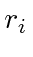and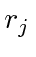must be transformed with a rotation matrix. Theis invariant since it is a dot product. So lets try the transformation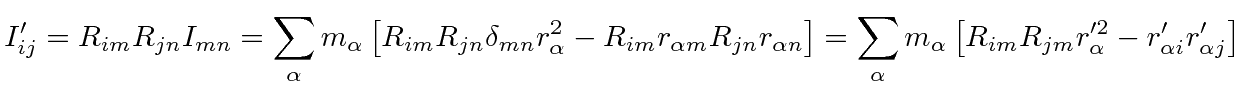This is the same formula for the inertia tensor written in terms of the primed coordinates, so this transformation leaves the formula for the inertia tensor invariant. We can see that a rank two tensor transforms with two rotation matrices, one for each index. We also saw this the identity tensorcan transform the same way but is actually invariant.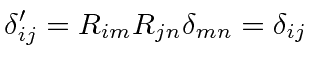Like a vector, a tensor is defined by how it transforms under rotations and parity inversion. All rank two tensors will transform the same way.

In summary, the inertia tensor transforms under rotations like any other rank 2 tensor.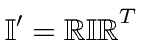Jim Branson 2012-10-21